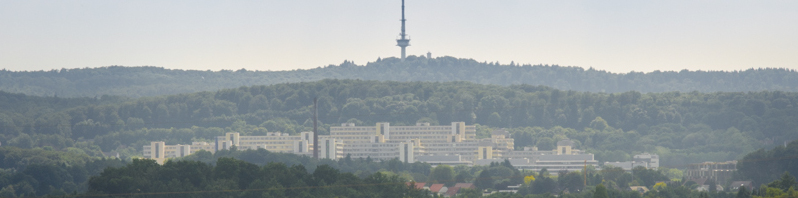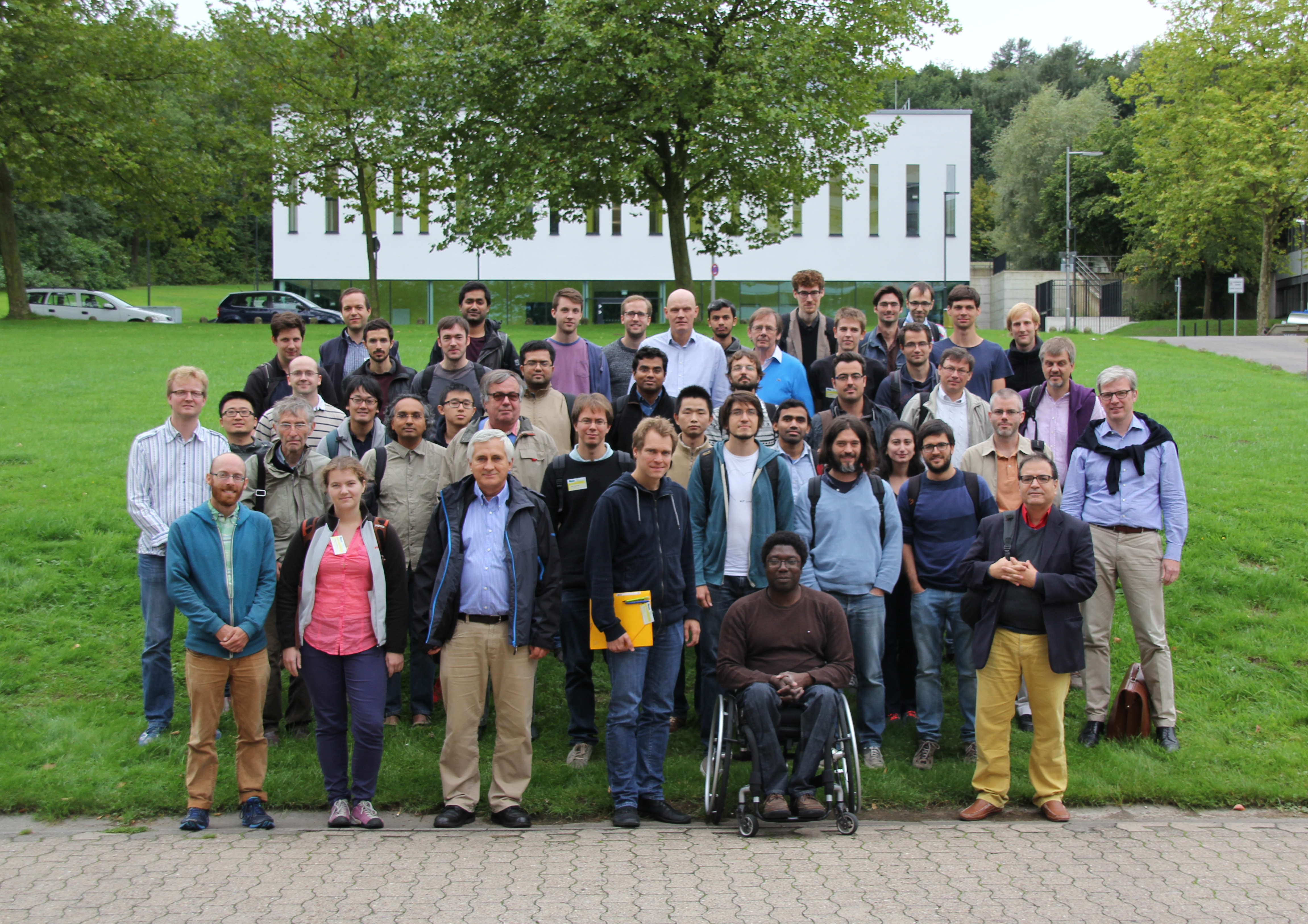Workshop "p-adic Hodge theory and Iwasawa theory"# p-adic Hodge theory and Iwasawa theory

The Faculty of Mathematics will host the workshop

p-adic Hodge theory and Iwasawa theory

September 14th — 18th, 2015

Bielefeld University

Conference PhotoThe participants of the 2015 p-adic Hodge theory and Iwasawa theory workshop.

## Invited Speakers

• Denis Benois (Bordeaux)
• Laurent Berger (Lyon)
• Veronika Ertl (Regensburg)
• Laurent Fargues (Jussieu)
• Lennart Gehrmann (Duisburg-Essen)
• Eugen Hellmann (Bonn)
• Fabian Januszewski (Karlsruhe)
• Henri Johnston (Exeter)
• Kiran Kedlaya (San Diego)
• Guido Kings (Regensburg)
• Farid Mokrane (Paris)
• Matthew Morrow (Bonn)
• Robert Pollack (Boston)
• Benjamin Schraen (CNRS)
• Otmar Venjakob (Heidelberg)
• Jean-Pierre Wintenberger (Strasbourg)
• Gergely Zábrádi (Budapest)

## Talks

All talks will be held in H6.

• Denis Benois (Bordeaux)
On $p$-adic height pairings
In the first part of this talk we generalize Nekovář’s construction of $p$-adic heights for Selmer complexes to the non-ordinary case. In the second part, we discuss some applications of this con- struction to the Iwasawa theory of modular forms (joint work in progress with Kazim Büyükboduk).
• The goal of this talk is to explain some recent results concerning Lubin-Tate $(\phi,\Gamma)$-modules'. As in the classical cyclotomic case, one can use them to construct cohomology classes, and then elements of the Iwasawa cohomology groups. I will review the relevant objects, the construction of cohomology classes, and give explicit formulas relating Bloch-Kato's exponential map and these classes. Joint work with L.~Fourquaux.
• I will discuss properties of the recently developed arithmetic syntomic cohomology due to Nekovář-Nizioł that generalises log-syntomic cohomology, and Besser's rigid syntomic cohomology, and lie out a comparison, using methods developed by Kurihara, with the goal of extending this comparison to more general cases. Part of what I will discuss is work in progress.
• I will speak about results contained in my article "$G$-torseurs en théorie de Hodge $p$-adique" linked to local class field theory. I will in particular explain the computation of the Brauer group of the curve and why its fundamental class is the one from local class field theory.
• Lennart Gehrmann (Duisburg-Essen)
Shalika models and $p$-adic $L$-functions
$p$-adic $L$-functions for cohomological cuspidal automorphic representations of $\mathrm{GL}(2n)$, which have a Shalika model, were first constructed by Ash and Ginzburg in the case of trivial coefficients. We will discuss a new, more conceptual construction, which works for arbitrary coefficients.
• We prove new classicality criteria for overconvergent $p$-adic automorphic forms of finite slope on definite unitary groups. These criteria are formulated in terms of the local geometry of a space parametrizing trianguline representations. Trianguline representations are a class of representations of the Galois group of a local $p$-adic field. By definition the $(\phi,\Gamma)$-module over the Robba ring associated to a trianguline representation admits a complete flag stable under $\phi$ and $\Gamma$. These representations naturally vary in rigid analytic families and may be seen as a counterpart of eigenvarieties. This is joint work with C. Breuil and B. Schraen.
• In the 90ies Clozel identified an important class of automorphic representations of $\mathrm{GL}(n)$, which conjecturally correspond to motives. This raises the question how rationality properties of motives are reflected on the automorphic side. In this talk I will discuss the existence of global rational structures on automorphic respresentations and present applications to special values of $L$-functions.
• The $p$-adic Stark conjecture at $s=1$' is formulated for a finite Galois extension of totally real number fields $L/K$ and a prime $p$. It relates the leading terms at $s=1$ of $p$-adic Artin $L$-functions to those of the complex Artin $L$-functions attached to $L/K$. When $L=K$ this is equivalent to Leopoldt's conjecture for $L$ at $p$ and the $p$-adic class number formula at $s = 1$' of Colmez. An important motivation for studying the $p$-adic Stark conjecture at $s = 1$ is that Burns and Venjakob have shown that it allows one to descend' from the equivariant Iwasawa main conjecture to deduce the relevant special case of the equivariant Tamagawa number conjecture (ETNC). We prove the $p$-adic Stark conjecture at $s=1$ for absolutely abelian extensions and use the representation theory of finite groups to reduce it to Leopoldt's conjecture for many non-abelian extensions. As a consequence, we obtain strong new evidence for certain cases of the ETNC. This is work in progress joint with Andreas Nickel.
• In the original evolution of the theory of $(\phi, \Gamma)$-modules out of the work of Fontaine, Colmez, Berger, et al., the base rings were certain rings of power series with $p$-adic coefficients. These rings carry certain Frobenius lifts which are injective but not surjective. By contrast, if one revisits this theory within the framework of perfectoid algebras, one gets a parallel theory in which the base rings have bijective Frobenius actions, and the role of the cyclotomic tower can be played by an arbitrary strictly APF extension. It is thus reasonable to ask to what extent constructions from the original theory can or cannot be replicated in the perfectoid setting; we will discuss some positive and negative answers to this question.
• In our joint work with Loeffler and Zerbes an explicit reciprocity law for Rankin-convolutions of modular forms was proved, which leads to new finiteness results on the Tate-Shafarevich group for elliptic curves. The strategy of the proof relies on using non-critical points of the $p$-adic $L$-function and a theory of $p$-adic interpolation of the étale realization of motivic Eisenstein classes via the so called Eisenstein-Iwasawa class. In this talk we explain our approach, discuss other cases where this strategy works and describe a new result, which generalizes the Eisenstein-Iwasawa classes.
• Given a modular form $f$ and a quadratic imaginary field $K$, one can form a $p$-adic $L$-function interpolating central critical values of the complex $L$-function of $f$ twisted by characters of the anticyclotomic $\mathbb{Z}_p$-extension of $K$. Under suitable parity conditions, one shows that this $p$-adic $L$-function is non-zero, and we can consider its $\mu$ and $\lambda$ Iwasawa invariants. When $f$ lives in a Hida family, we show that these invariants are constants on branches, obtaining an anticyclotomic analogue of a similar result by Emerton-Pollack-Weston in the cyclotomic setting. This result allows us to spread results on the main conjecture from one form to all other forms in the family, obtaining new cases of the anticyclotomic main conjecture. This is a joint work with F. Castella and C.-H. Kim.
• The classical Hodge-Tate map of the connected $p$-divisible group of the universal oridinary abelian variety extends to a strict neighborhood of the ordinary locus and allows us to interpolate Siegel modular forms when the weight varies. I will give some elements of the proof of these facts, these will be related to toroidal compactifications and Mumford quotients. This is joint work with Olivier Brinon and Jacques Tilouine.
• I will speak about the construction of a new integral $p$-adic cohomology theory interpolating crystalline and étale, obtained by glueing de Rham-Witt complexes and the pro-étale cohomology of period sheaves. This is joint work with B. Bhatt and P. Scholze.
• In this talk, we present a new conjecture on slopes of $p$-adic modular forms. We write down a relatively simple and explicit power series over weight space and conjecture, in the $\Gamma_0(N)$-regular case, that this power series knows the slopes of the $U_p$ operator acting on all spaces of overconvergent modular forms. Precisely, we conjecture that the Newton polygon of our series evaluated at a weight $k$ (classical or not) matches the Newton polygon of the characteristic power series of $U_p$ acting on weight $k$ overconvergent modular forms. We call this power series the ghost series'' as its spectral curve hovers around the true spectral curve.

In this talk, we will explain this ghost conjecture and its connections to other conjectures on slopes (e.g.~Buzzard's conjecture, the Gouvea-Mazur conjecture, Coleman's spectral halo) and discuss implications for the shape and structure of the eigencurve.

This is a joint project with John Bergdall.

• I'll explain how to prove the existence of an infinitesimal character on the locally analytic vectors of certain $p$-adic Banach representations appearing as Hecke eigenspaces in some completed cohomology spaces. I'll draw some consequences concerning the canonical dimension of these $p$-adic Banach representations.
• We generalize work of Fontaine and Herr about expressing local Galois cohomology and Iwasawa cohomology in terms of $(\phi,\Gamma)$-modules from the cyclotomic case to the case of Lubin-Tate extensions. In particular we show a kind of reciprocity law which admits to calculate the image of an adequate Kummer map in the first Iwasawa cohomology group. This is joint work with Peter Schneider (Münster).
• Jean-Pierre Wintenberger (Strasbourg)
Ramification and Iwasawa modules
We give criteria for Leopoldt's conjecture and we construct by modular means a Galois representation whose ramification satisfies most of the properties of the criteria. (jw C. Khare)
• Let $G$ be the group of $\mathbb{Q}_p$-points on a $\mathbb{Q}_p$-split connected reductive group with connected centre. There are (at least) two candidates (one by Breuil and the other by Schneider and Vignéras) for the generalization of Colmez's "Montréal functor" (from the case $G=\mathrm{GL}_2(\mathbb{Q}_p)$ to this generality) adjoining a (generalized) étale $(\phi,\Gamma)$-module to a smooth mod $p^n$ representation $\pi$ of $G$. In the talk I will show how to obtain Breuil's $(\phi,\Gamma)$-module by a series of taking étale hull, localisation, and pseudocompact completion of the Schneider-Vignéras functor. Moreover, assuming certain nonvanishing of a variant of Breuil's functor we show how to realize the Pontryagin dual of $\pi$ as a $G$-invariant subspace in the global sections of a $G$-equivariant sheaf on the flag variety $G/B$ where $B$ is a Borel subgroup. This is joint work with M. Erdélyi.

## Contact

In case of questions feel free to contact Andreas Nickel.

name@math.uni-bielefeld.de; name: anickel3